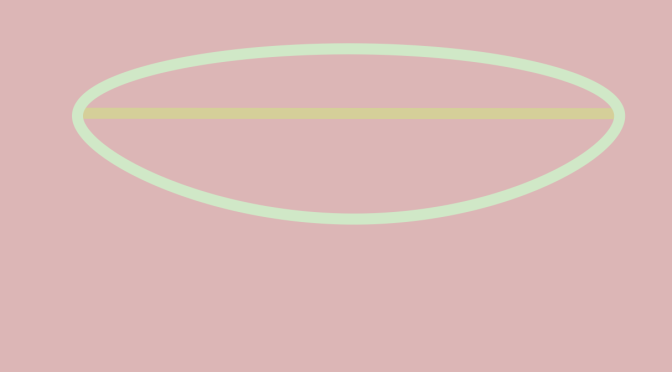# Counterexamples around connected spaces

A connected space is a topological space that cannot be represented as the union of two or more disjoint nonempty open subsets. We look here at unions and intersections of connected spaces.

### Union of connected spaces

The union of two connected spaces $$A$$ and $$B$$ might not be connected “as shown” by two disconnected open disks on the plane.

However if the intersection $$A \cap B$$ is not empty then $$A \cup B$$ is connected.
The intersection of two connected spaces $$A$$ and $$B$$ might also not be connected. An example is provided in the plane $$\mathbb R^2$$ by taking for $$A$$ the circle centered at the origin with radius equal to $$1$$ and for $$B$$ the segment $$\{(x,0) \ : \ x \in [-1,1]\}$$. The intersection $$A \cap B = \{(-1,0),(1,0)\}$$ is the union of two points which is not connected.